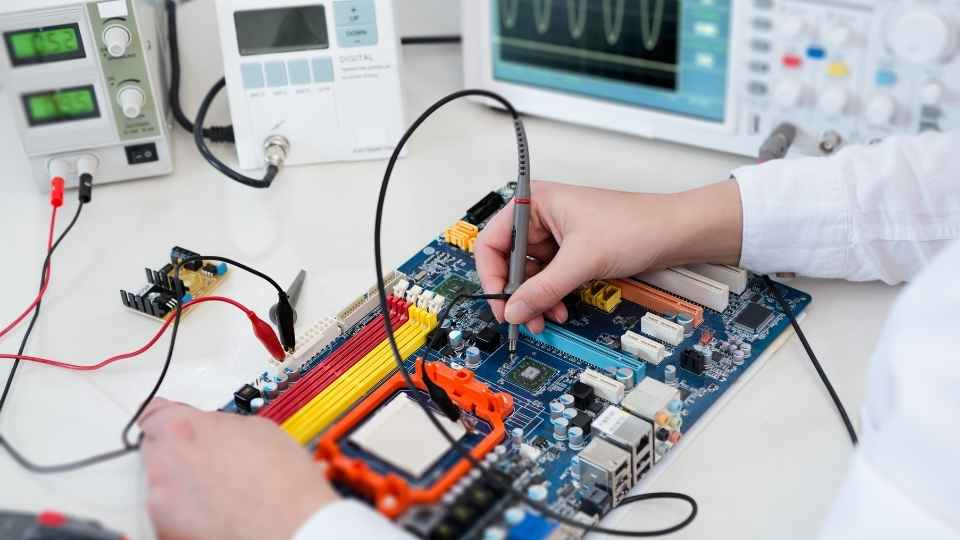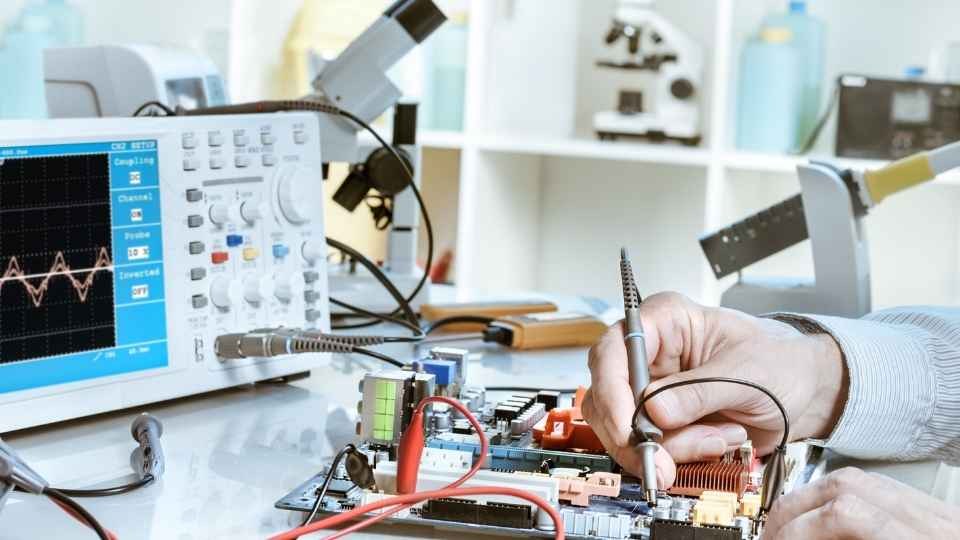# Introduction to Electronic Theory: Demystifying Basic ConceptsAre you baffled by the complexities of electronic theory? Do terms like circuit analysis, Ohm's Law, and component behavior leave you feeling overwhelmed? Fear not!

In this article, we will demystify these basic concepts and provide you with a clear understanding of electronic theory. Whether you're a novice or an experienced professional looking to refresh your knowledge, our technical and precise explanations will guide you through the fundamentals.

Get ready to unlock the secrets of electronic theory and gain the freedom to explore this fascinating field with confidence.

## Key Takeaways

• Electronic theory explores the behavior and flow of electrons in materials and circuits.
• Voltage, current, resistance, capacitance, and inductance are fundamental concepts in electronic theory.
• Circuit analysis techniques such as Kirchhoff's Laws, Ohm's Law, Node Analysis, Mesh Analysis, and Superposition Theorem are used to study electrical circuits.
• Understanding component behavior, such as resistors, capacitors, and inductors, is crucial in electronic systems.

## The Fundamentals of Electronic Theory

The fundamentals of electronic theory lay the groundwork for understanding the principles and mechanisms behind the functioning of electronic devices. At its core, electronic theory explores the behavior and flow of electrons in various materials and circuits. It encompasses concepts such as voltage, current, resistance, capacitance, and inductance.

Voltage refers to the electric potential difference between two points in a circuit, while current is the flow of electric charge through a conductor. Resistance opposes this flow and is determined by factors like material properties and physical dimensions. Capacitance measures an object's ability to store electrical energy, whereas inductance pertains to a component's ability to generate an electromagnetic field when current flows through it.

## Understanding Circuit Analysis

Circuit analysis is a fundamental aspect of understanding electronic systems. It allows us to study the behavior and properties of electrical circuits, enabling us to design, troubleshoot, and optimize electronic devices. To demystify this concept further, here are some key points:

• Kirchhoff's Laws: These laws describe the conservation of charge and energy in an electrical circuit.• Ohm's Law: This law states that the current flowing through a conductor is directly proportional to the voltage applied across it.

• Node Analysis: A technique used to determine voltages at different nodes in a circuit by applying Kirchhoff's Current Law.

• Mesh Analysis: This method involves applying Kirchhoff's Voltage Law to determine currents in different loops of a circuit.

• Superposition Theorem: This theorem simplifies complex circuits by analyzing them individually under different sources.

Understanding these concepts empowers individuals with the freedom to comprehend and manipulate electronic systems for their desired outcomes.

## Exploring Component Behavior

Exploring various components' behavior provides valuable insights into the intricate workings of electronic systems, allowing for informed design decisions and optimized performance. Understanding how individual components, such as resistors, capacitors, and inductors, behave within a circuit is crucial for engineers to ensure the desired functionality of the system.

For instance, by studying resistor behavior, one can determine its resistance value and power dissipation capabilities. Capacitors exhibit characteristics like capacitance and voltage dependency that influence their charging and discharging behaviors. Inductors store energy in magnetic fields and exhibit properties like inductance and reactance.## An In-Depth Look at Ohm's Law

Ohm's Law, a fundamental principle in electrical engineering, relates the voltage across a resistor to the current flowing through it and the resistance of the component. Understanding Ohm's Law is crucial for analyzing and designing electronic circuits. Here are some key points to consider:

• Voltage (V): The potential difference across a component, measured in volts (V).

• Current (I): The flow of electric charge through a component, measured in amperes (A).

• Resistance (R): The opposition to the flow of current offered by a component, measured in ohms (Ω).

• Ohm's Law Equation: V = I * R

• Power (P): The rate at which work is done or energy is transferred, calculated using P = V * I.

## Demystifying Basic Electronic Concepts

Understanding the fundamental principles of electricity is essential for engineers and individuals working in the field of electrical engineering. To demystify basic electronic concepts, it is crucial to grasp key terms and ideas related to this subject matter.One of the primary concepts in electronics is voltage, which refers to the electric potential difference between two points in a circuit. Current, on the other hand, represents the flow of electrons through a conductor. Resistance is another critical concept that measures how much an object or material opposes the flow of current.

In addition to these fundamental concepts, it is vital to understand power and energy in electronics. Power relates to how quickly work can be done or how fast energy is transferred. Energy, however, refers to the capacity of a system or component to do work.

### How Do Electronic Circuits Affect the Environment and What Measures Can Be Taken to Minimize Their Impact?

Electronic circuits can have a significant impact on the environment due to their production, energy consumption, and waste generation. To minimize their impact, measures such as using energy-efficient components, recycling electronic waste, and adopting sustainable manufacturing practices should be implemented.

### What Are the Common Challenges Faced by Beginners When Learning Electronic Theory and How Can They Be Overcome?

Beginners in electronic theory often face challenges such as understanding complex concepts, troubleshooting circuit issues, and acquiring practical skills. These can be overcome through comprehensive study materials, hands-on experimentation, and seeking guidance from experienced professionals.

### Can Electronic Theory Be Applied to Other Fields Outside of Electrical Engineering?

Yes, electronic theory can be applied to various fields outside of electrical engineering. It is a fundamental knowledge base that can be utilized in areas such as telecommunications, computer science, robotics, and even biomedical engineering.

### What Are Some Practical Applications of Ohm's Law in Everyday Life?

Ohm's law, a fundamental principle in electrical engineering, finds practical applications in everyday life. It is used to calculate the current flowing through different components of electrical circuits and helps in designing and troubleshooting electronic devices.

Yes, there are several emerging trends and advancements in electronic theory that readers should be aware of. These include quantum computing, artificial intelligence, internet of things (IoT), and 5G technology. Stay informed to keep up with the latest developments in this field.